# Train a Perceptron to Learn the AND Gate from Scratch in Python

## The Representative Power of a Perceptron

##### So, a perceptron shown below, is capable of learning decision boundaries in a binary classification scenario. For example, if your inputs are 1-Dimensional or 2-Dimensional, we can actually visualize different parts of our perceptron. Below, in the 1-Dimensional case you can see how the input and the bias unit are linearly combined using the weights, resulting in Z. Then you can see that we apply the thresholding function on top of Z to create our binary classifier. This classifier outputs +1 for instances of one class, and -1 for the instances of the other class.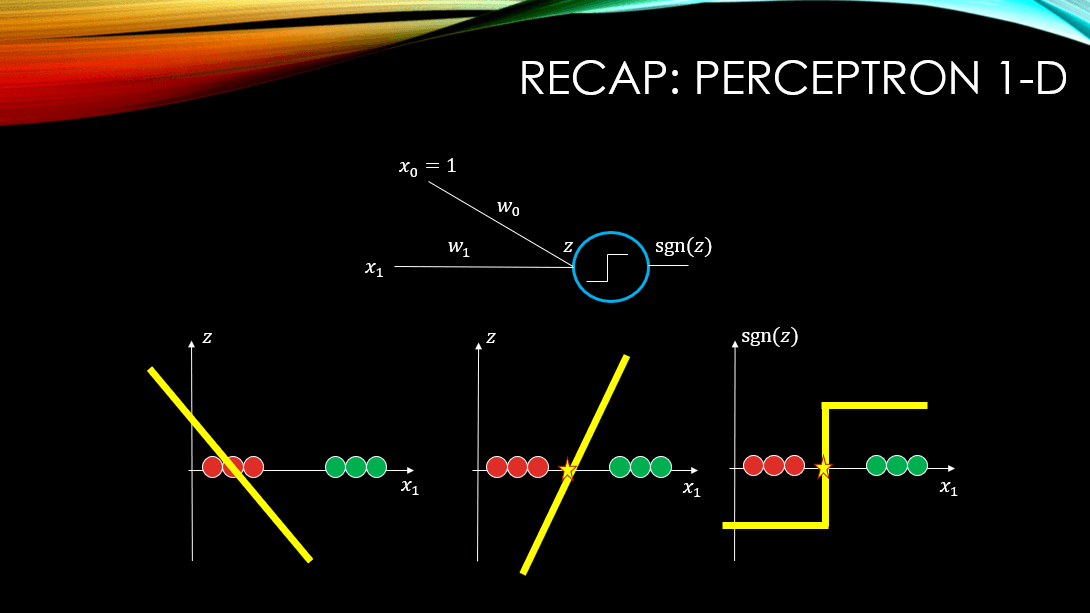##### The sgn(Z) is our sign function where if Z>0, outputs +1 and if Z<0, it outputs -1. You notice how when our input data is 1-Dimensional, our Z is a 2-Dimensional figure namely a line:. However, our decision boundary, where Z cuts through our input space (i.e., where Z=0), is a point and it is 1-Dimensional just like our input data. What about a 2-Dimensional input data? What will Z be then?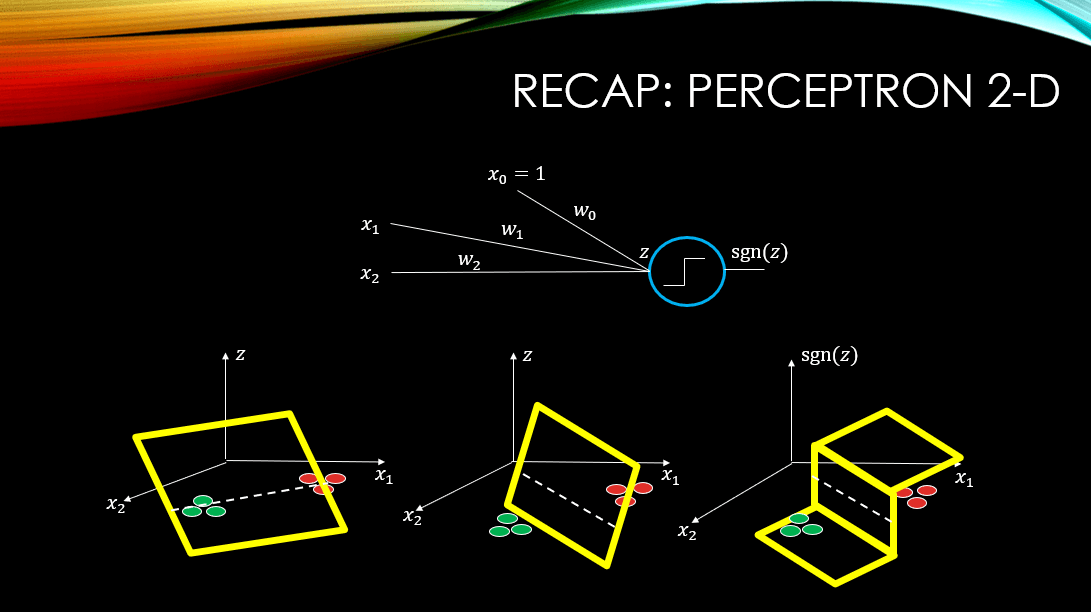### Boolean Functions (A Reminder)

##### So, boolean functions, at least the main ones can be summarized, below: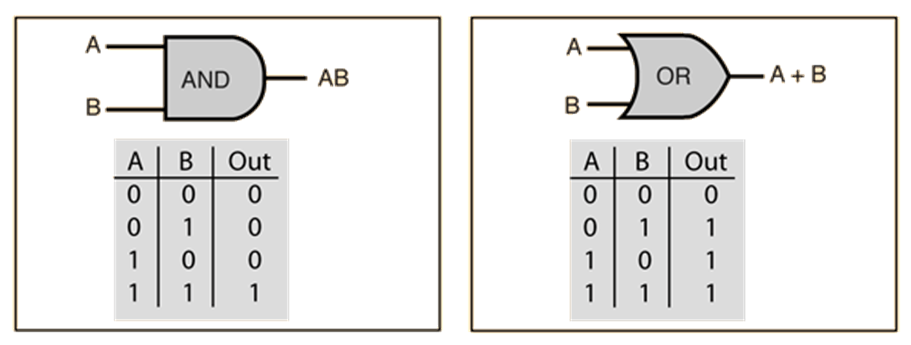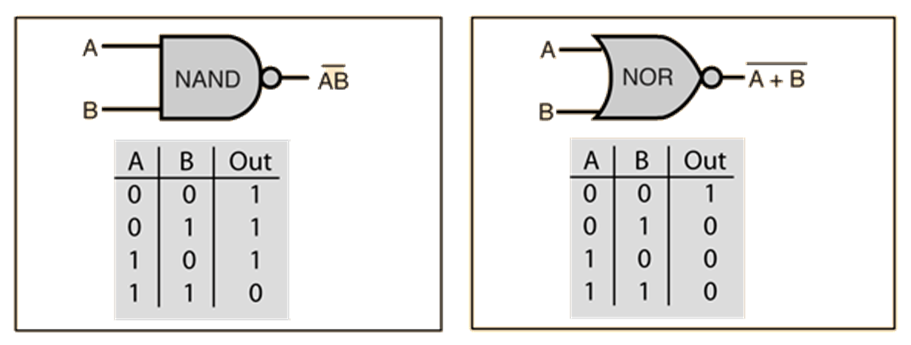## Linearly Separable Gates and Perceptrons

##### If we look at the 2-Dimensional input space of boolean functions, and color positive examples with green and negatives with red, then we can visualise our training data like below. Note that, the yellow line is a decision boundary that can separate the 2 classes beautifully, and hopefully our neural network will be able to find such a boundary!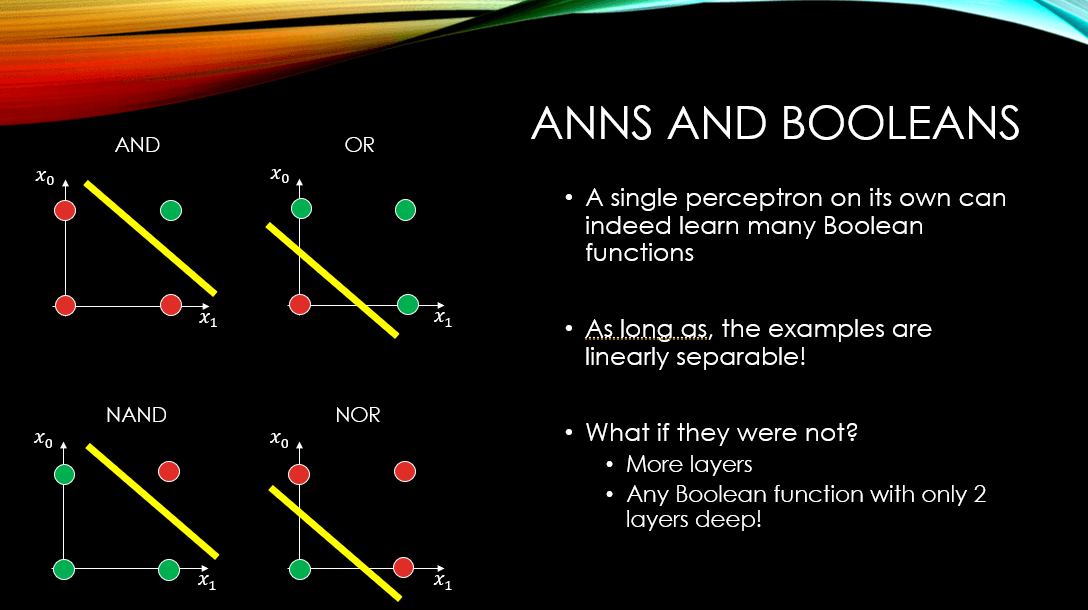##### It is important to note that not all gates can be learned by a single perceptron! For instance the XOR gate, represented below, can NEVER EVER be learned by a single perceptron! Why?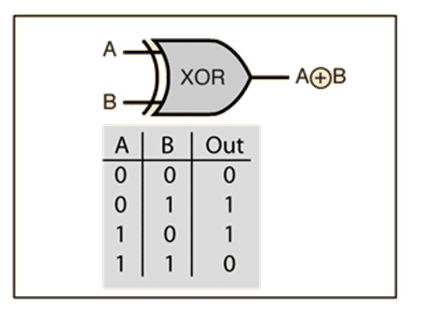##### Now what about the XOR?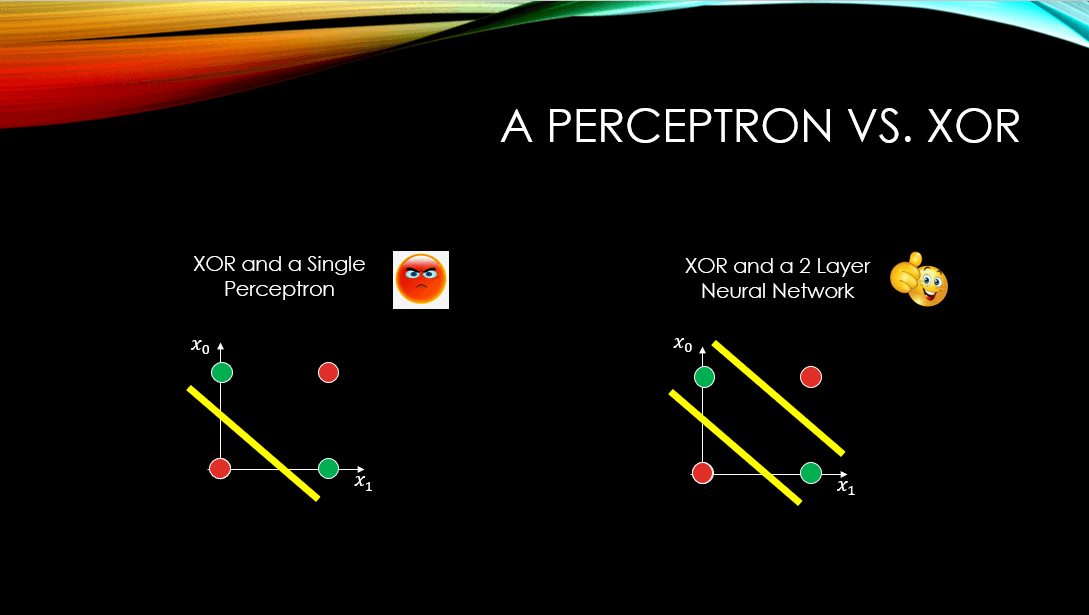## Teaching a Perceptron the AND Gate (CODE)

##### Of all the gates that are linearly separable, we have chosen the AND gate to code from scratch. First foremost, let’s take a look at our perceptron: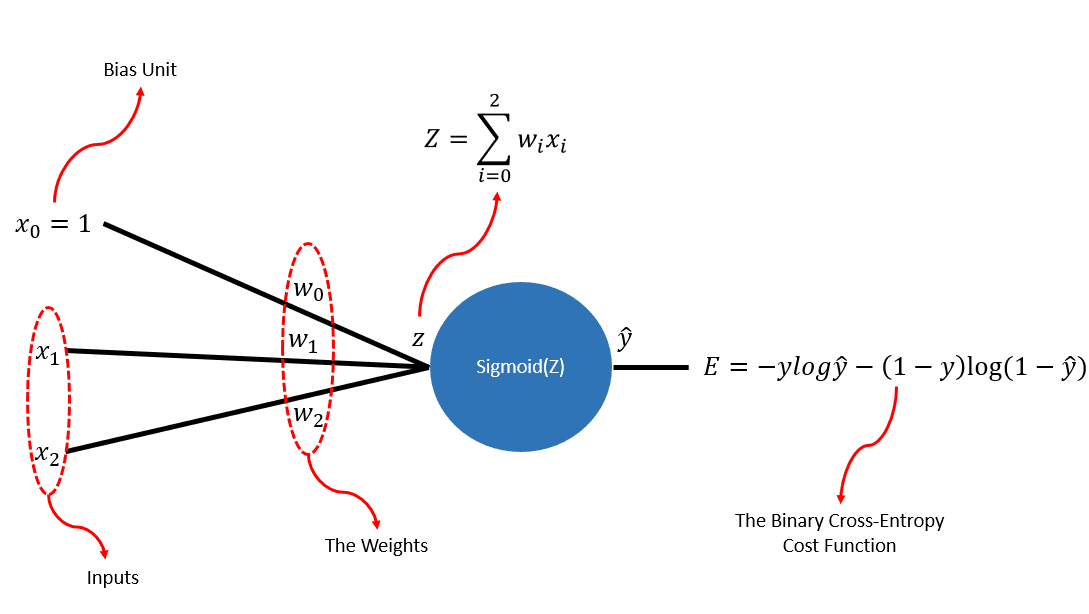##### Let’s also visualise our dataset using the following code: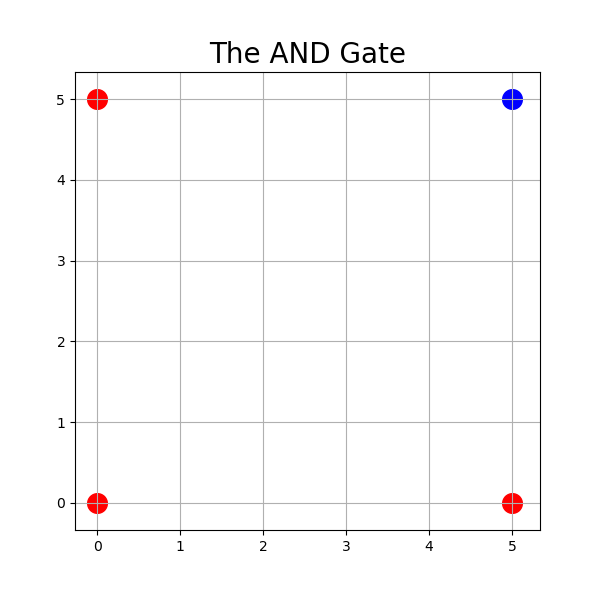##### Here is one of the outputs generated after 500 epochs: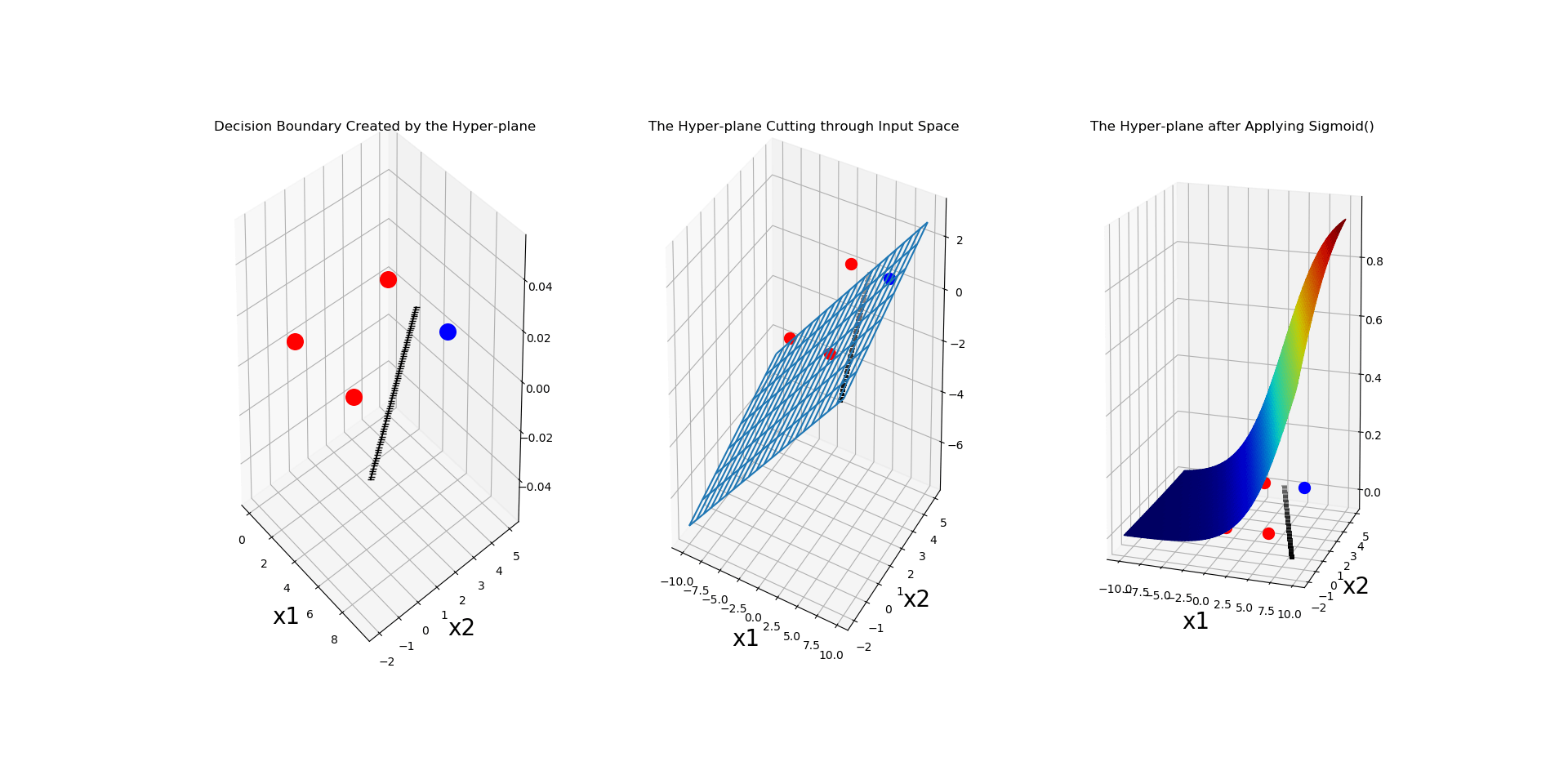##### And let’s also plot the error values that we have been recording, per epoch: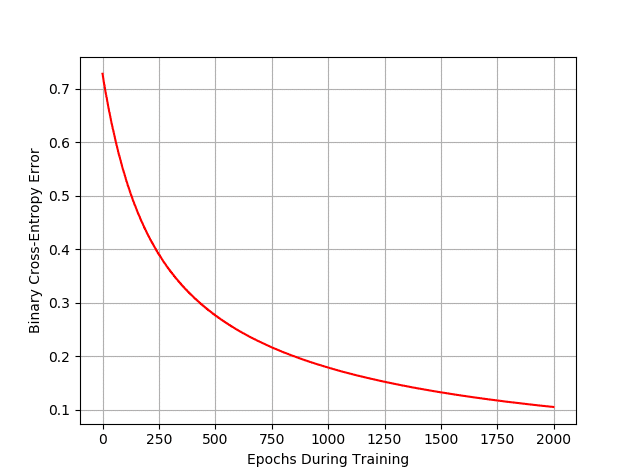## I Have 2 Challenges for You !!!

##### The network should NEVER converge as there is no way for a line to separate linearly inseparable examples! The error function should look something nasty and non-converging like this: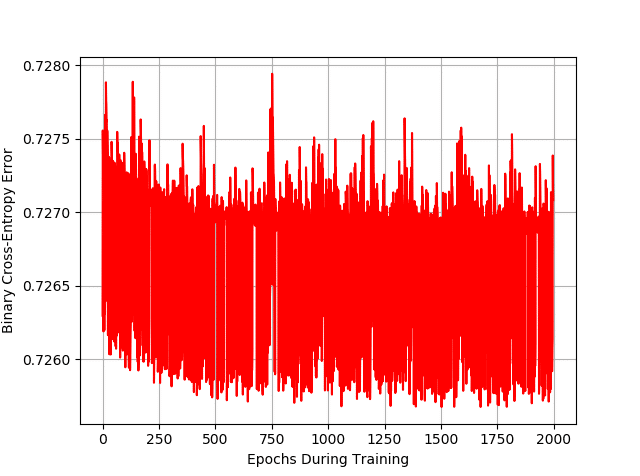### 2 thoughts on “Train a Perceptron to Learn the AND Gate from Scratch in Python”

1.Excellent explanation

2.I need a code of learning perceptron or gate using step function